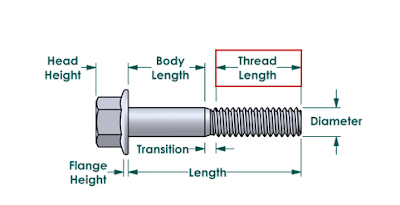# How to Measure the Size of a Bolt ?A bolt’s size is classified based on a number of dimensions, namely the bolt’s diameter, length of shank, thread pitch, grade, thread length and head size. Bolts can me specified in metric using millimeters, but the standard, internationally-recognized descriptions of bolt sizes are in inches.

Measuring a bolt: the tools you’ll need
Are you measuring metric bolts or standard bolts? Standard bolt sizes are slightly different and are measured in inches while metric bolts are measured in millimeters (mm).
Otherwise, bolt measurements are carried out the same way, except when it involves threads, which we’ll get to.

For measuring bolts with accuracy, you’ll find these tools helpful:

Caliper:
You’ll need this for measuring metric thread pitch. Standard uses threads per inch and doesn’t require a caliper for that task, but it’s helpful for determining if thread on a bolt is straight or tapered.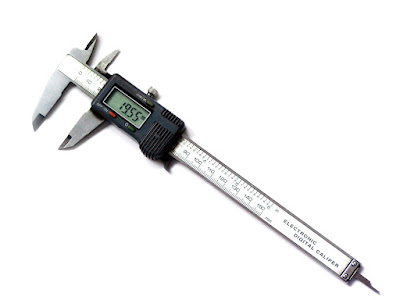Bolt size gauge: A handy tool that lets you fit bolts through bolt-sized holes. The bolt gauge is divided into standard and metric sections. Simply push the bolt into the holes on the bolt gauge and find the smallest hole the bolt will fit into and you’ll have the size you need.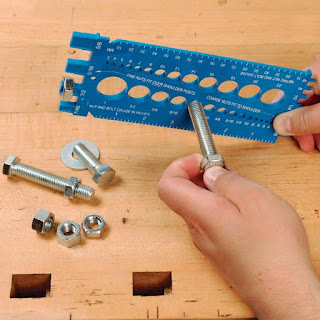If you don’t have either tool at hand, you can use a ruler for measuring bolts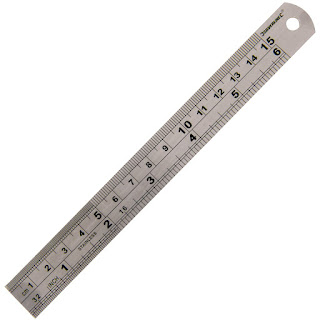If you’re looking to figure out the size of a bolt needed in an assembly and have a bolt that has worked on hand, follow the steps below to identify the dimensions of the bolt:

Step 1: Measure the Shank’s Diameter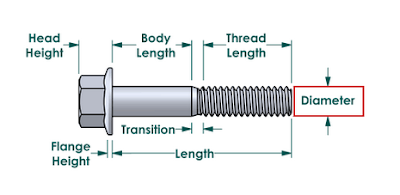The shaft of the bolt is called the shank, and its diameter is the first dimension used to describe a bolt size. This can be done using calipers or a bolt gauge.

Step 2: Determine the Thread Pitch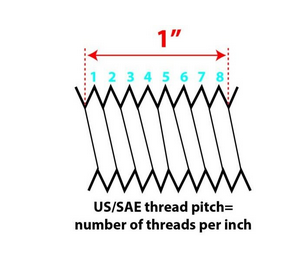Thread pitch is a designation related to the number of threads per inch on the bolt’s shank. You can complete this measurement by simply counting the number of threads in an inch worth of shank. If the shank is less than one inch, you’ll need to multiply the number of threads to reach a full inch worth of threading.

Step 3: Measure the Length of the Shank
This is the measurement from where the shank meets the head to the very tip of the fastener.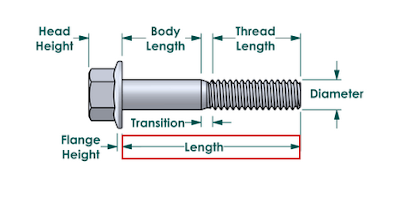Step 4: Determine the Bolt’s Grade
The grade of a bolt is determined by the type of metal used in the manufacturing of the bolt, as indicated by the bolt’s head markings. No one expects you to know all of the head markings by heart, just use our handy bolt head marking chart to make the determination.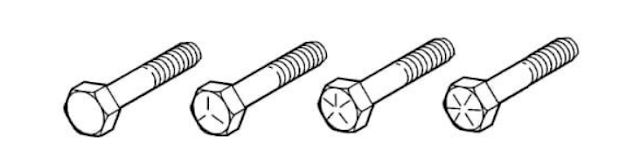Step 5: Determine the Shape of the Bolt’s Head
This should be a simple one. While the most common bolt head is hex (six-sided), some applications require a four-sided square head or the dome-shaped head of a carriage bolt.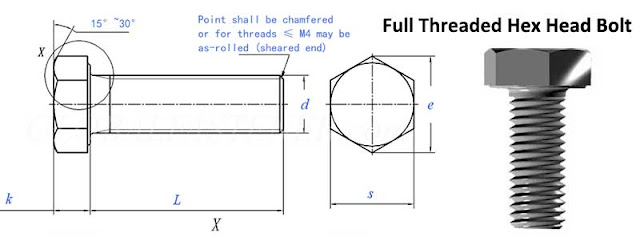Step 6: Measure the Length of the Threaded Portion of the Bolt
As opposed to the shank length measured in Step 3, this measurement should specify only the length of the threaded portion of the bolt. One instance in which this would be useful to know is when determining whether you need a standard hex bolt or rather a fully threaded tap bolt.